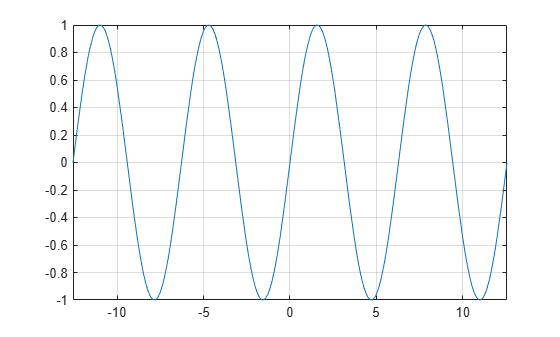# sin

Symbolic sine function

## Syntax

``sin(X)``

## Description

example

``sin(X)` returns the sine function of `X`.`

## Examples

### Sine Function for Numeric and Symbolic Arguments

Depending on its arguments, `sin` returns floating-point or exact symbolic results.

Compute the sine function for these numbers. Because these numbers are not symbolic objects, `sin` returns floating-point results.

`A = sin([-2, -pi, pi/6, 5*pi/7, 11])`
```A = -0.9093 -0.0000 0.5000 0.7818 -1.0000```

Compute the sine function for the numbers converted to symbolic objects. For many symbolic (exact) numbers, `sin` returns unresolved symbolic calls.

`symA = sin(sym([-2, -pi, pi/6, 5*pi/7, 11]))`
```symA = [ -sin(2), 0, 1/2, sin((2*pi)/7), sin(11)]```

Use `vpa` to approximate symbolic results with floating-point numbers:

`vpa(symA)`
```ans = [ -0.90929742682568169539601986591174,... 0,... 0.5,... 0.78183148246802980870844452667406,... -0.99999020655070345705156489902552]```

### Plot Sine Function

Plot the sine function on the interval from $-4\pi$ to $4\pi$.

```syms x fplot(sin(x),[-4*pi 4*pi]) grid on```### Handle Expressions Containing Sine Function

Many functions, such as `diff`, `int`, `taylor`, and `rewrite`, can handle expressions containing `sin`.

Find the first and second derivatives of the sine function:

```syms x diff(sin(x), x) diff(sin(x), x, x)```
```ans = cos(x) ans = -sin(x)```

Find the indefinite integral of the sine function:

`int(sin(x), x)`
```ans = -cos(x)```

Find the Taylor series expansion of `sin(x)`:

`taylor(sin(x), x)`
```ans = x^5/120 - x^3/6 + x```

Rewrite the sine function in terms of the exponential function:

`rewrite(sin(x), 'exp')`
```ans = (exp(-x*1i)*1i)/2 - (exp(x*1i)*1i)/2```

### Evaluate Units with `sin` Function

`sin` numerically evaluates these units automatically: `radian`, `degree`, `arcmin`, `arcsec`, and `revolution`.

Show this behavior by finding the sine of `x` degrees and `2` radians.

```u = symunit; syms x f = [x*u.degree 2*u.radian]; sinf = sin(f)```
```sinf = [ sin((pi*x)/180), sin(2)]```

You can calculate `sinf` by substituting for `x` using `subs` and then using `double` or `vpa`.

## Input Arguments

collapse all

Input, specified as a symbolic number, scalar variable, matrix variable, expression, function, matrix function, or as a vector or matrix of symbolic numbers, scalar variables, expressions, or functions.

collapse all

### Sine Function

The sine of an angle, α, defined with reference to a right triangle isThe sine of a complex argument, α, is

`$\mathrm{sin}\left(\alpha \right)=\frac{{e}^{i\alpha }-{e}^{-i\alpha }}{2i}\text{\hspace{0.17em}}.$`

## Version History

Introduced before R2006a

expand all